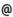## "Babes-Bolyai" University of Cluj-Napoca Faculty of Mathematics and Computer Science

 Functional analysis (1)
 Code Semes-ter Hours: C+S+L Credits Type Section MO004 5 2+2+0 6 compulsory Matematică MO004 5 2+2+0 5 compulsory Matematică-Informatică
 Teaching Staff in Charge
 Lect. BRECKNER Brigitte Erika, Ph.D., brigittemath.ubbcluj.ro
 Prof. BRECKNER Wolfgang, Ph.D., brecknermath.ubbcluj.ro
 Lect. TRIF Tiberiu Vasile, Ph.D., ttrifmath.ubbcluj.ro
 Aims Presentation of the fundamental notions and results of functional analysis.
 Content 1. Complements of linear spaces theory (4 hours course + 4 hours exercise classes) Linear spaces, linear subspaces, linear hull. Linear operators, linear functionals, the correspondence between complex-linear functionals and the real-linear ones. Sublinear maps and halfnorms. The extension of linear functionals (the Hahn-Banach theorem, the Bohnenblust-Sobczyk-Suhomlinov theorem). 2. Linear topological spaces (6 hours course + 14 hours exercise classes) The notion of a linear topological space. The interior and the adherence of a set in a topological linear space. Multihalfnormed spaces. The normed spaces B(T,K), CB(T,K), C(T,K), l_\infty, c, c_0, l_p. Summable families of points of a normed space. 3. Prehilbertian spaces (6 hours course + 2 hours exercise classes) The notion of a prehilbertian space. Hilbert spaces. Orthogonality. The problem of the best approximation, the orthogonal decomposition theorem for prehilbertian spaces. Orthonormed families, the computation of the best approximation points, the Gram-Schmidt orthonormalization procedure. Orthonormed bases. 4. Continuous linear operators (6 hours course + 4 hours exercise classes) Characterisations of the continuity of linear operators on multihalfnormed spaces. The open operator theorem. The closed graph theorem. The normed space of continuous linear operators on normed spaces. The singularities condensation principle, the uniform boundedness principle. The pointwise convergence of sequences of continuous linear operators. The divergence of the Lagrange interpolation sequence. The solving of linear equations using the succesively approximation method, the C. Neumann series associated to a continuous linear operator, the C. Neumann theorem. Topological isomorphisms between normed spaces. 5. Continuous linear functionals (6 hours course + 4 hours exercise classes) Continuous linear functionals on multihalfnormed spaces. Continuous linear functionals on normed spaces, the Hahn theorems. The general form of continuous linear functionals on the normed spaces l_p, c, c_0. The adjoint of a linear continuous operator. Reflexive normed spaces. The algebraical-topological dual of a Hilbert space.
 References 1. CONWAY J.B.: A Course in Functional Analysis. Second Edition. Springer-Verlag, New York - Berlin - Heidelberg, 1990. 2. HEUSER H.: Funktionalanalysis. Theorie und Anwendung. 3. Auflage. B. G. Teubner, Stuttgart, 1992 3. KANTOROVICI L.V., AKILOV G. P.: Analiză funcţională. Editura Stiintifica si Enciclopedica, Bucuresti, 1986 4. MATHIEU M.: Funktionalanalysis. Ein Arbeitsbuch. Spektrum Akademischer Verlag, Heidelberg - Berlin, 1998 5. MUNTEAN I.: Analiza functionala. Universitatea "Babes-Bolyai", Cluj-Napoca, 1993 6. POPA E.: Culegere de probleme de analiza functionala. Editura Didactica si Pedagogica, Bucuresti, 1981 7. WERNER D.: Funktionalanalysis. Vierte, ueberarbeitete Auflage. Springer-Verlag, Berlin - Heidelberg - New York, 2002
 Assessment A mid-term written examination and an end-term viva voce examination.